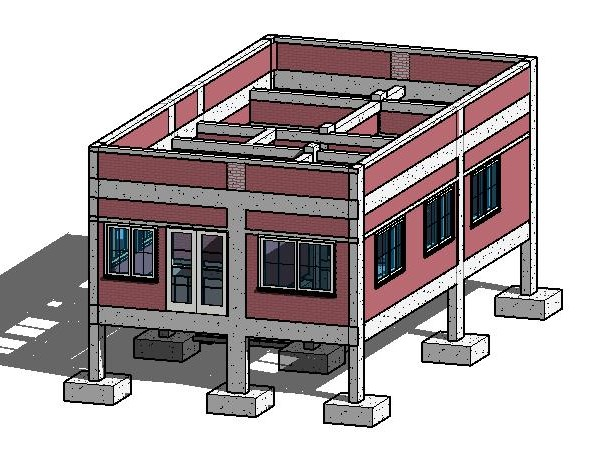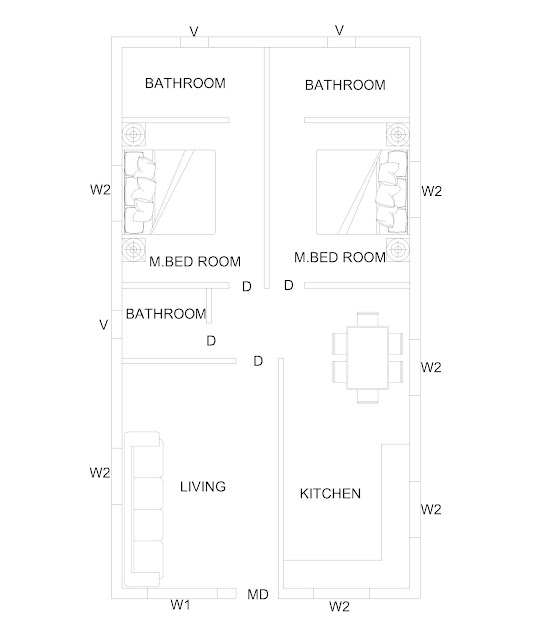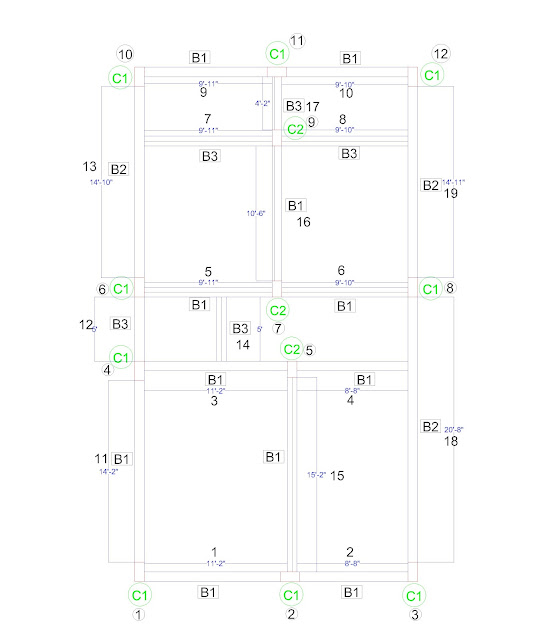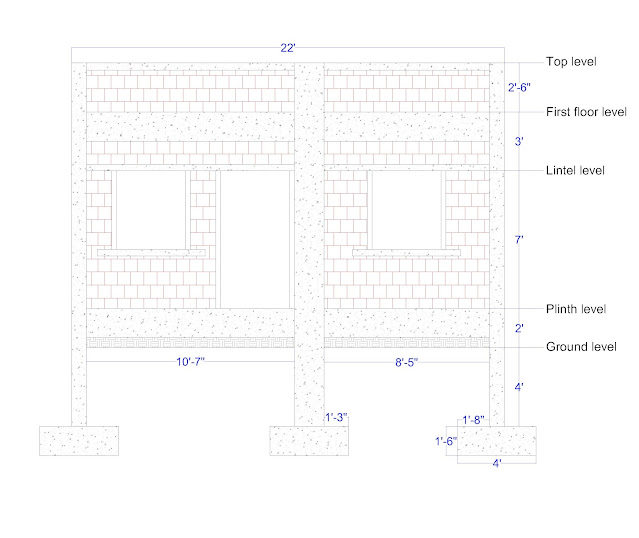# CONSTRUCION COST PER SQUARE FEET OF A HOUSE

It is very essential to calculate the construction cost of a house for making budget or arrange finance, as construction cost is increasing day by day.

I have made a complete series regarding learning how to calculate the construction cost of a house per square feet. Also, in last I will upload a excel sheet of construction estimate, you have to just update the new rates and measurements and you will get the cost of your house.

Today, we will discuss about the total construction cost of a g+1 floor house.

We divide into four parts for calculating the construction cost of a house.

## Understanding the stages involves in construction of house

There are several parts of a building house, 1- RCC stage, 2- Finishing Stage, 3-Decor stage,4-Handover stage.

#### 1- RCC stage

contains Excavation, Back filling, Concreting(beam-columns, slab, lintel, chajjas, footing, coping, etc.), shattering, BBS, Curing, etc.

#### 2- finishing stage

contains masonry, plaster, color work, electrical wiring, plumbing, fabrication, glass work, door-window fittings, flooring, false ceiling, kitchen point, dedo tiles, etc.

#### 3- Décor stage

contains plantation, furniture, wallpaper, decorative paints, curtain, etc.

#### 4- Handover stage

means the testing of a house for living, it include some of minor works or some remaining revision works to be done.

## Construction cost of RCC work (material)

Before calculations we need all the relative drawings and information regarding the house.

Here are some data regarding construction,

Grade of concrete - M20 (1:1.5:3)

Grade of PCC - M15 (1:2:4)

Sand - local fine sand

Grade of mortar-     1:4 and 1:6

Plaster thickness -   20 mm external walls

; 10 mm internal walls

Thickness of walls- 9 inch outer wall

; 4.5 inch partition wall

Here are drawings for the calculations of construction cost of house.3D view
You can see 3D design of the house, which we will calculate the construction cost.Architectural plan

Two master bedroom, kitchen and living room with common bathroom are provided in this plan.Beam-columns layout plan
Here you can see beam column layout plan with the dimensions.Section-plan
This is the front side section plan of the house.
You can see a vertical dimensions of each level. Also, every columns contains the footing.
Size of structural members are given in below table.

 Sr. No. Beam No Length Breadth Width 1 B1 Varies 9 18 2 B2 Varies 9 15 3 B3 Varies 9 12 4 C1 Varies 9 18 5 C2 Varies 9 15 6 Coping 1 Varies 9 4.5 7 Coping 2 Varies 4.5 4.5 8 Lintel 1 Varies 9 4.5 9 Lintel 2 Varies 4.5 4.5 10 Footing 4’ 4’ 1’6” height 11 Main Door 7’ - 4’ height 12 Internal door 7’ - 3’ height 13 W1 4’ - 5’ height 14 W2 4’ - 4’ height 15 V 2’ - 2’ height

We will start numbering from bottom left to right and left bottom to top for beam and column you can see that numbering in beam column layout plan.

Before calculating the construction cost of a house we need quantities of various structural members like beam, columns, slabs, footing, lintels, coping, PCC, etc.

### Column quantity

We have 18.5 feet height and width of  9 inch means 9/12= 0.75 feet breadth of 18/12 = 1.5 feet.
We will multiply 0.75x1.5x18.5 and we will get 20.8 cum. Ft.
We will do the same calculation for all the columns.

After calculating all the columns we are getting 239.3 cum. ft. volume of the concrete in columns only.
We will convert it on to cum. m. for ease of calculation.

### Beam quantity

For beams, the calculation is same as beam is also rectangular in shape.
In the case of beam, we will consider in to in distance for finding the length of the beam.
You can see B1-1 beam is 11.16 feet long and cross sectional dimensions are 9 x 18 inch means 0.75 x 1.5.

Volume for B1-1 is 11.16x0.75x1.5 = 12.56 cum. ft.
After finding all the length we need a final quantity of concrete used in the all beams.

I have provided Ground beam and first floor beam with same dimensions for ease of calculations.
So, for all beams we have quantity of concrete is 222.31 x 2 = 444.62 cum. ft.

### Footing quantity

We have footing size of 4'x4'x1.5' = 24 cum. ft.

We generally provide lintels at 7 feet from plinth level, 9 inch lintel is used in the outer wall and 4.5 inch thick lintel used in the all internal walls.

Sometimes coping is provided below the window for screwing purpose or some other purpose.
In our case we will consider lump sump of 12 cum. ft. concrete in coping.
Also in parapet wall we will provide coping.

### PCC quantity calculations

PCC means plain cement concrete. We don't use reinforcement in PCC. PCC can not beam tensile load, as steel rebar is not used on it.

In footing bed we use PCC, Thickness of PCC is 4 Inch and 6 inch bigger than footing in all the directions.
So we will get PCC of 5 x 5 x 0.25 feet which is  6.25 cum. ft.

We have 12 footings So total PCC will be 12 x 6.25 = 75 cum. ft. which is 2.16 cum. m.
In ground/plinth slab we also use PCC. So, we get total 4.95 cum. m. of M15 grade PCC slab at the plinth level.

Now the total 2.16+4.95 = 7.11  of  PCC work to be done.

### Steel quantity calculations

For calculating steel in the structural members, we use thumb rule for now.

As we know the density of steel is 7850 kg/m3. which means in 1 m3 of steel weight is 7850 kg.
For columns, we take 2.5% steel.
So,  2.5% of 6.75 m3 of concrete in the case of columns.
Steel in columns= (2.5x7850x6.75)/100 = 1324.68 kg

In the case of beams, we will take 2% of  12.6m3,
So, steel in beam = (2x7850x12.6)/100= 1978.2 kg

For slab, we will take 1%.
Steel in slab = (1x7850x4.95)/100=388.57 kg

For footings, we will take 0.8%.
Steel in footing = (0.8x7850x8.61)/100=540.71 kg

For lintels = (2x7850x2.46)/100 = 386.22 kg
Total steel = 1324.68+1978.2+388.57+540.71+386.22= 4618.38 kg
We will consider 5000 kg steel (wastage, additional work) for this house.

After this calculations, we have below values.

Concrete in columns - 6.78 cum. m.
Concrete in beams - 12.6 cum. m.
Concrete in slabs - 4.95 cum. m.
Concrete in footings - 8.61 cum. m.
Concrete in lintels & coping (M15) - 2.46 cum. m.
PCC(M15)- 2.16 cum. m.
PCC slab(M15) - 4.95 cum. m.

Concrete = 6.78+12.6+4.95+8.61= 32.94 m3
We will need total 32.94 m3 M20 grade concrete.
In M20 (1:1.5:3) grade concrete, 8.22 bags cement, 616.5 kg sand and 1233 kg aggregate is used.
So in our case we will need,

Cement = 8.22 x 32.94 = 271 bags
Sand = 616.5 x 32.94 = 20308 kg
Aggregate = 1233 x 32.94 =  40615 kg

We need, 2.46+2.16+4.95 = 9.57 m3 of M15 grade concrete.
In M15 (1:2:4) grade concrete, 6.34 bags cement, 634 kg sand and 1268 kg aggregate is used.

Cement =  6.34 x 9.57 = 61 bags
Sand = 634 x 9.57 = 6068 kg
Aggregate = 1268 x 9.57 = 12135 kg.

Now, we have material cost of RCC structure from rates and quantity of various items.
Material rates varies from place to place and time to time, currently I am using the rates of Gujarat region.

For 1 ton of steel we will take 10 kg of binding wire and 10 kg nail as per past experience.
We don't consider the shattering material cost because it will be in the scope of the contractor.

 Unit Volume Rate Total Cement (Bags) 332 420 1,39,440 ₹ Sand (ton) 26.380 650 17,150 ₹ Aggregate (ton) 52.750 580 30,600 ₹ Steel (kg) 5000 72 3,60,000 ₹ Binding wire (kg) 50 80 4,000 ₹ Nail (kg) 50 80 4,000 ₹ Total - - 5,55,190 ₹

## Conclusion

From the above calculations and discussion we can say that construction cost material of RCC of supposed house is 5,55,190 INR.
We have 22' x 40' feet which is 880 square feet house. So, construction cost per square feet will be 555190/880 = 630.9 INR.
We have two slabs in this plan (G+1) , In some region people provide the rates according to slabs.
So, in our case the construction cost per square feet is 316 INR.

After quantity calculations and material rate calculation we will discuss about the labor rates for RCC construction in the next article.